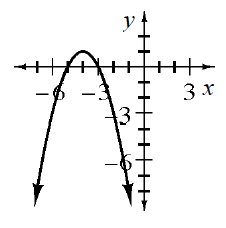### Home > INT2 > Chapter 10 > Lesson 10.2.3 > Problem10-87

10-87.

For the function $f(x) = -(x + 4)^2 + 1$:

1. Sketch a graph of the function.2. Where is the function increasing?

Where are $y$ values increasing and to which point?

3. What is the maximum value of the function?

The maximum value is the greatest $y$-value.